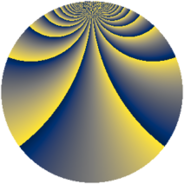# Properties

 Label 2880.2.dyLevel $2880$ Weight $2$ Character orbit 2880.dy Rep. character $\chi_{2880}(109,\cdot)$ Character field $\Q(\zeta_{16})$ Dimension $1904$ Sturm bound $1152$

# Learn more about

## Defining parameters

 Level: $$N$$ $$=$$ $$2880 = 2^{6} \cdot 3^{2} \cdot 5$$ Weight: $$k$$ $$=$$ $$2$$ Character orbit: $$[\chi]$$ $$=$$ 2880.dy (of order $$16$$ and degree $$8$$) Character conductor: $$\operatorname{cond}(\chi)$$ $$=$$ $$320$$ Character field: $$\Q(\zeta_{16})$$ Sturm bound: $$1152$$

## Dimensions

The following table gives the dimensions of various subspaces of $$M_{2}(2880, [\chi])$$.

Total New Old
Modular forms 4672 1936 2736
Cusp forms 4544 1904 2640
Eisenstein series 128 32 96

## Trace form

 $$1904q - 16q^{4} + 8q^{5} + O(q^{10})$$ $$1904q - 16q^{4} + 8q^{5} - 8q^{10} + 16q^{11} + 16q^{14} - 16q^{16} - 16q^{19} + 8q^{20} - 8q^{25} - 64q^{26} + 16q^{29} - 16q^{34} + 8q^{35} + 32q^{40} + 16q^{41} + 16q^{44} - 16q^{46} - 16q^{49} + 32q^{50} + 56q^{55} - 80q^{56} - 48q^{59} - 16q^{61} - 112q^{64} + 16q^{65} - 104q^{70} + 80q^{71} + 112q^{74} - 48q^{76} + 16q^{79} + 32q^{80} - 8q^{85} + 16q^{86} + 16q^{89} - 16q^{91} + 160q^{94} + O(q^{100})$$

## Decomposition of $$S_{2}^{\mathrm{new}}(2880, [\chi])$$ into newform subspaces

The newforms in this space have not yet been added to the LMFDB.

## Decomposition of $$S_{2}^{\mathrm{old}}(2880, [\chi])$$ into lower level spaces

$$S_{2}^{\mathrm{old}}(2880, [\chi]) \cong$$ $$S_{2}^{\mathrm{new}}(320, [\chi])$$$$^{\oplus 3}$$$$\oplus$$$$S_{2}^{\mathrm{new}}(960, [\chi])$$$$^{\oplus 2}$$